×#### Thank you for registering.

One of our academic counsellors will contact you within 1 working day.

Click to Chat

1800-1023-196

+91-120-4616500

CART 0

• 0

MY CART (5)

Use Coupon: CART20 and get 20% off on all online Study Material

ITEM
DETAILS
MRP
DISCOUNT
FINAL PRICE
Total Price: Rs.

There are no items in this cart.
Continue Shopping• Complete Physics Course - Class 11
• OFFERED PRICE: Rs. 2,968
• View Details

```Emf and Grouping of cells

Table of Content

Electromagnetic Force and Potential Difference

Internal Resistance of a Cell

Grouping of Cells

Problem (JEE Main)

Related Resources

Electromagnetic Force and Potential Difference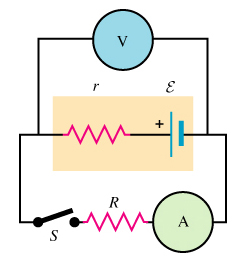Emf is an extremely important term associated with a cell. When we talk about any circuit, there are certain components in the circuit which insert energy into the circuit while certain components withdraw energy. The device which puts energy into the circuit is said to be providing an electromotive force while those withdrawing energy from the circuit are said to be exhibiting potential difference across them. Potential difference is abbreviated as ‘pd’ and emf is an abbreviation for electromotive force. Both these terms are measured in volts and they illustrate the quantity of energy inserted or drawn out per coulomb of charge passing through the specific portion of the circuit.

Emf of a cell can also be defined as the work performed or required for transforming a unit positive charge from negative to positive terminal within the cell. Though its nature resembles that of a force, but it should not be confused with force in literal sense. In simple scientific words, we may defined emf and pd as:Potential difference – It is also abbreviated as pd and is the quantity of electrical energy that is transformed into some other forms of energy per unit of charge.

Emf – It is the amount of energy in any form which is transformed into electrical energy per coulomb of charge.

Sources of emf – Emf is generally generated in nature whenever some kinds of variations occur along a surface. Some of the major sources of emf include:

cell, battery (a combination of cells), solar cell, voltaic cells, generator, dynamo, thermocouple, transformers.

When a conductor is placed in a varying magnetic field, it results in the production of emf in the system. This kind of emf is called as induced emf.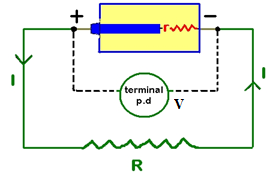Figure shows a source of currents that is, a cell connected to a conductor of resistance R through a key K. A voltmeter V is connected across the two terminals of the cell.

When the key K is open, the reading of voltmeter gives the electromotive force (em.f) E of the cell.

The electromotive force E of a cell is defined as the difference of potential between its terminals when there is no current in the external circuit. That is, when the cell is in open circuit.

When the key is closed, an electric current i flows from positive to negative terminal in the external circuit and from negative terminal to positive terminal within the cell. Thus, a potential drop ‘r’ takes place across the internal resistance ‘r’ (shown separately) of the cell. The direction of ‘ir’ is opposite to the e.m.f. ‘E’ of the cell. The reading of the voltmeter now decreases and gives the potential difference V between the terminals when it is in closed circuit.

So, V = E – ir

Or, E = V + ir

Hence, E is greater than V by an amount equal to the drop of potential within the cell due to its internal resistance.

Internal Resistance of a Cell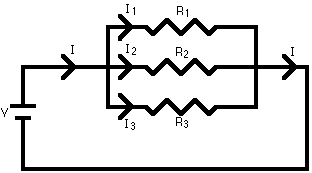In an electric circuit, the current starts from the positive terminal of a cell and enters the negative terminal after passing through the circuit. In order to maintain the continuity, the current has to pass through the electrolyte of the cell from negative terminal to the positive terminal.

The resistance offered by the electrolyte of the cell when the electric current passes through it is known as the internal resistance of the cell. It is denoted by r.

Now, consider circuit diagrams as shown in figure.

If ‘E’ and ‘V’ are the readings of voltmeter when the key ‘K’ is open and closed respectively. That is, if E represents e.m.f. and V the potential difference of the cell, then

V = E – ir     …... (1)

Since, i = e.m.f./resistance

Thus, i = E/R+r    (Since, R and r are in series with each other)

Putting this value of ‘i’ in equation (1), we get,

V = E – [(E/R+r).r]

V = E [1 – (r/R+r)]

V = E (R+r-r/R+r)

V = E (R/R+r)

Thus, V (R+r) = ER

Or, VR + vr = ER

Or,Vr = ER – VR = R (E – V)

Thus, r = R (E – V/V)

Knowing E, V and R, the value of ‘r’ can be calculated.

Grouping of Cells

If a large number of cells are available for sending current through a load, the best combination will be that which sends maximum current. The type of combination depends upon the value of load resistance:

(a) Cells in series: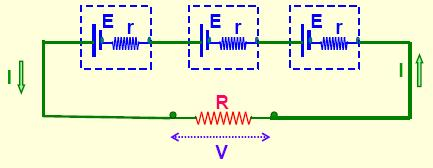Cells are said to be connected in series if negative terminal of first is connected with positive terminal of the second and so on figure.

Consider n cells each of e.m.f. E and internal resistance r connected across the two terminals of an external resistance R.

Total e.m.f. of the combination = nE

Total resistance of the circuit = R + nr

Current ‘i’ in the circuit is given by i = total e.m.f./total resistance

i = nE/R+nr

Case (i). If R <<nr,     i = NE/nr = E/r

This current is independent of ‘n’. Therefore, there is no increase in current due to an increase in number of cells. For such a load this type of combination is not useful.

Case (ii). If R >> nr,      i = nE/R

Current increases with an increase in n. So, grouping of cells in series is useful if the external resistance is much larger than the total internal resistance of the combination.

(b) Cells in parallelCells are said to be connected in parallel if the positive terminals of all the cells are connected at one place and the negative at the other. The combination sends a current through an external resistance R as shown in figure.

Let ‘E’ be the e.m.f. and ‘r’ the internal resistance of each cell. Since all the cells are connected in between two common terminals,

Total e.m.f. of the combination = E

If R' is the total internal resistance of the combination,

1/R' is the total internal resistance of the combination,

1/R' = 1/r + 1/r + 1/r + … m times

So, 1/R' = m/r

So, R' = r/m

Total resistance of the circuit = R+R' = R+(r/m)

Therefore current ‘i’ flowing in the circuit is,

i = E/[R+(r/m)]

Case (i), If R » r/m

i = E/r

Since ‘i’ is independent of the number of  cells, the combination is not useful.

Case (ii). If R « r/m

i = E/(r/m) = m (E/r)

Current ‘i’ increases with an increase in the number of cells. So, combination of cells in parallel is useful when the external resistance is much smaller than the total resistance of the combination.

(c) Mixed grouping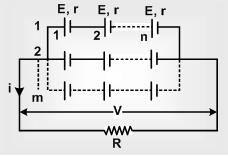Mixed grouping of cells consists of a number of cells connected in series with each other in one row and a number of such rows connected in parallel with each other.

Let n be number of cells in one row and m such rows are connected in parallel with each other as shown in figure.

If ‘E’ is the e.m.f. of each cell, then,

total e.m.f. of the combination = nE

If ‘r’ is the internal resistance of each cell, resistance of one row = nr

Total internal resistance R' of the combination is given by,

1/R' = 1/nr + 1/nr + …. m times

1/R' = m/nr

So, R' = nr/m

Total resistance of the circuit = R +  R' = R+(nr/m)

So, Current, i = nE/(R+nr/m) = mnE/(mR+nr)

Or i = nE/mR + nr

Here N = mn is the total number of cells. Combination is most useful if it sends maximum current through R. Current is maximum if the denominator ‘mR+nr’ is minimum.

mR + nr =  (√mR)2 + (√nr)2

=  (√mR)2 +  (√nr)2 – 2√mnRr + 2√mnRr

= [√(mR – nr)]2 + 2√mnRr

(mR + nr) is minimum if,

√mR – √nr = 0

Or, if mR = nr

That is, if R = nr/m

The mixed combination is most suitable if the cells are arranged in such a manner that the external resistance is equal to the internal resistance of the combination.

Refer this video to know more about grouping of cellsIf same current flows through a number of resistances connected to each other, they are in series with each other. In this case potential difference across different resistances will be different.

If the current gets divided into branches and is summed up back after passing through the resistances, they are in parallel with each other. In this case potential difference across different resistances is same.

On connecting a low resistance across (in parallel) any resistance results in a decrease of resistance while connecting a high resistance across it does not make appreciable difference.

On connecting a low resistance in series with a resistance does not make an appreciable difference while connecting a high resistance in series increases the resultant resistances appreciably.

In series combination,

Same current flows through each of the resistors.

Each resistor have their own corresponding voltage drop.

In parallel combination,

The potential difference across all the resistors are same.

Each resistor have it’s own individual current flowing through it which is given by Ohm’s law.

The total current flowing through the circuit is the sum of current flowing through each resistor.

Problem (JEE Main)

A cell develops the same power across two resistance R1 and R2 separately. The internal resistance of the cell is

(a) R1 + R2

(b) R1 + R2 / 2

(c) √(R1R2)

(d) [√(R1R2)] / 2

Solution

Let r be the internal resistance of the cell and E its EMF. When connected across the resistance R1 in the circuit, current passing through the resistance is

i = E/R1 + r

So, P1 = i2R1 = [E/(R1+r)]2R1

Similarly P2 = [E/(R2 +r)]2 R2

Given that P1 = P2

Substituting the values, we get,

r = √(R1R2)

From the above observation, we conclude that, option (c) is correct.Question 1

When n resistances each of value r are connected in parallel, then resultant resistance is x. When these n resistances are connected in series, total resistance is

(A) nx

(B) rnx

(C) x / n

(D) n2 x.

Question 2

Resistance of a wire is r ohms. The wire is stretched to double its length, then its resistance in ohms is

(A) r / 2

(B) 4 r

(C) 2 r

(D) r / 4.

Question 3

Which method can be used for absolute measurement of resistances ?

(A)Ohm's law method

(B)Wheatstone bridge method

(C)Raleigh method

(D) Lorentz method.

Question 4

Three 3 ohm resistors are connected to form a triangle. What is the resistance between any two of the corners?

(A) 3 / 4 ohms

(B) 3 ohms

(C) 2 ohms

(D) 4/3 ohm.

Question 5

How many different combinations may be obtained with three resistors, each having the resistance R ?

(A) 3

(B) 4

(C) 5

(D) 6.Q.1
Q.2
Q.3
Q.4
Q.5

d

b

b

c

b

Related Resources

You might like to Electric Current and Curent Density.

For getting an idea of the type of questions asked, refer the  Previous Year Question Papers.

To read more, Buy study materials of Current Electricity comprising study notes, revision notes, video lectures, previous year solved questions etc. Also browse for more study materials on Physics here.
```### Course Features

• 101 Video Lectures
• Revision Notes
• Previous Year Papers
• Mind Map
• Study Planner
• NCERT Solutions
• Discussion Forum
• Test paper with Video Solution